# 4.2   Sorting and Searching

The sorting problem is to rearrange an array of items in ascending order. In this section, we will consider in detail two classical algorithms for sorting and searching—binary search and mergesort—along with several applications where their efficiency plays a critical role.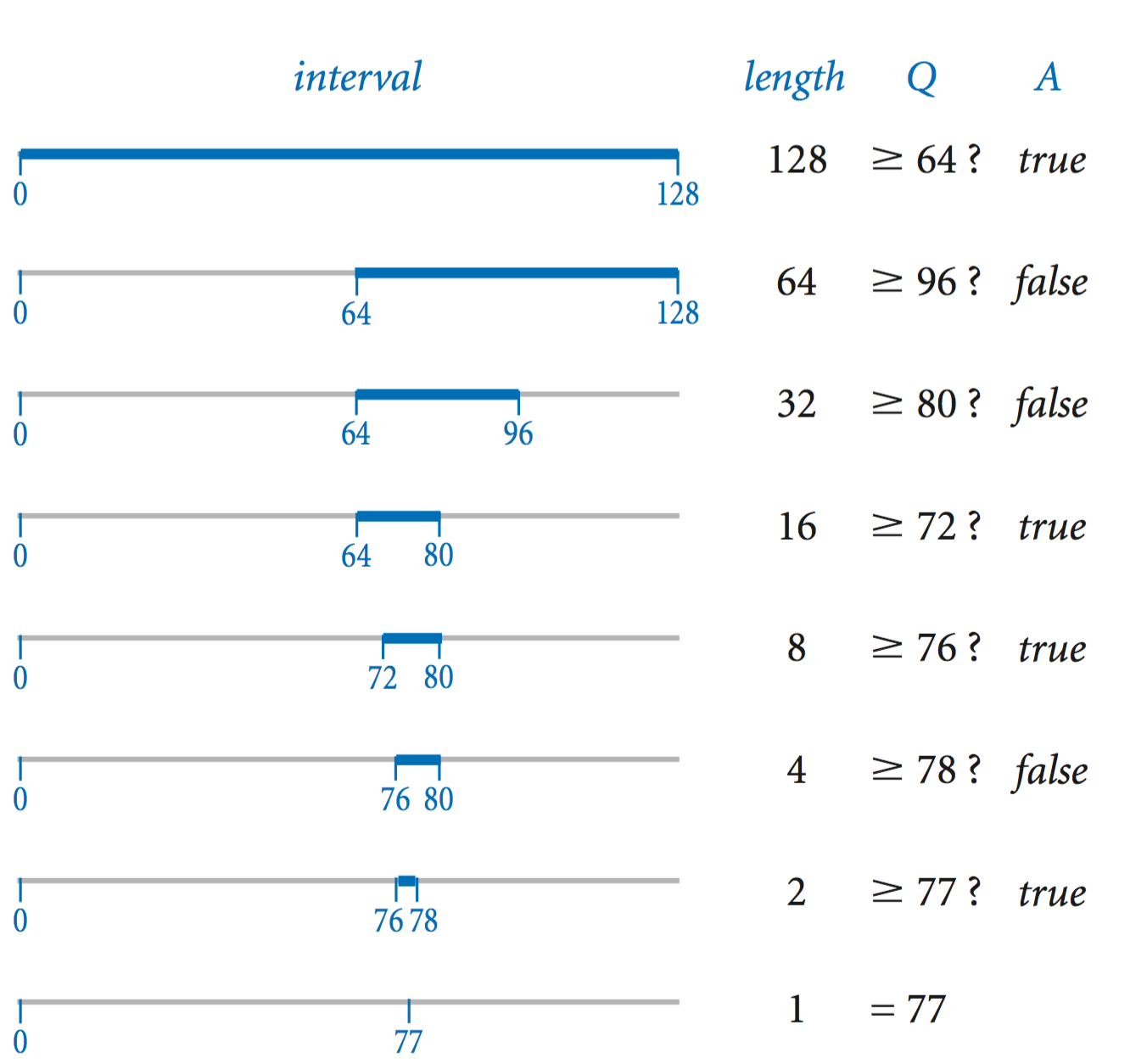## Binary search.

In the game of "twenty questions", your task is to guess the value of a secret number that is one of the n integers between 0 and n−1. For simplicity, we will assume that n is a power of 2 and that the questions are of the form "is the number greater than or equal to x?"

An effective strategy is to maintain an interval that contains the secret number, guess the number in the middle of the interval, and then use the answer to halve the interval size. Questions.java implements this strategy. It is an example of the general problem-solving method known as binary search.

• Analysis of running time. Since the size of the interval decreases by a factor of 2 at each iteration (and the base case is reached when n = 1), the running time of binary search is lg n.
• Linear–logarithm chasm. The alternative to using binary search is to guess 0, then 1, then 2, then 3, and so forth, until hitting the secret number. We refer to such an algorithm as a brute-force algorithm: it seems to get the job done, but without much regard to the cost (which might prevent it from actually getting the job done for large problems). In the worst case, the running time can be as much as n.
• Binary representation. If you look back to Binary.java, you will recognize that binary search is nearly the same computation as converting a number to binary! Each guess determines one bit of the answer. For example, if the number is 77, the sequence of answers no yes yes no no yes no immediately yields 1001101, the binary representation of 77.
•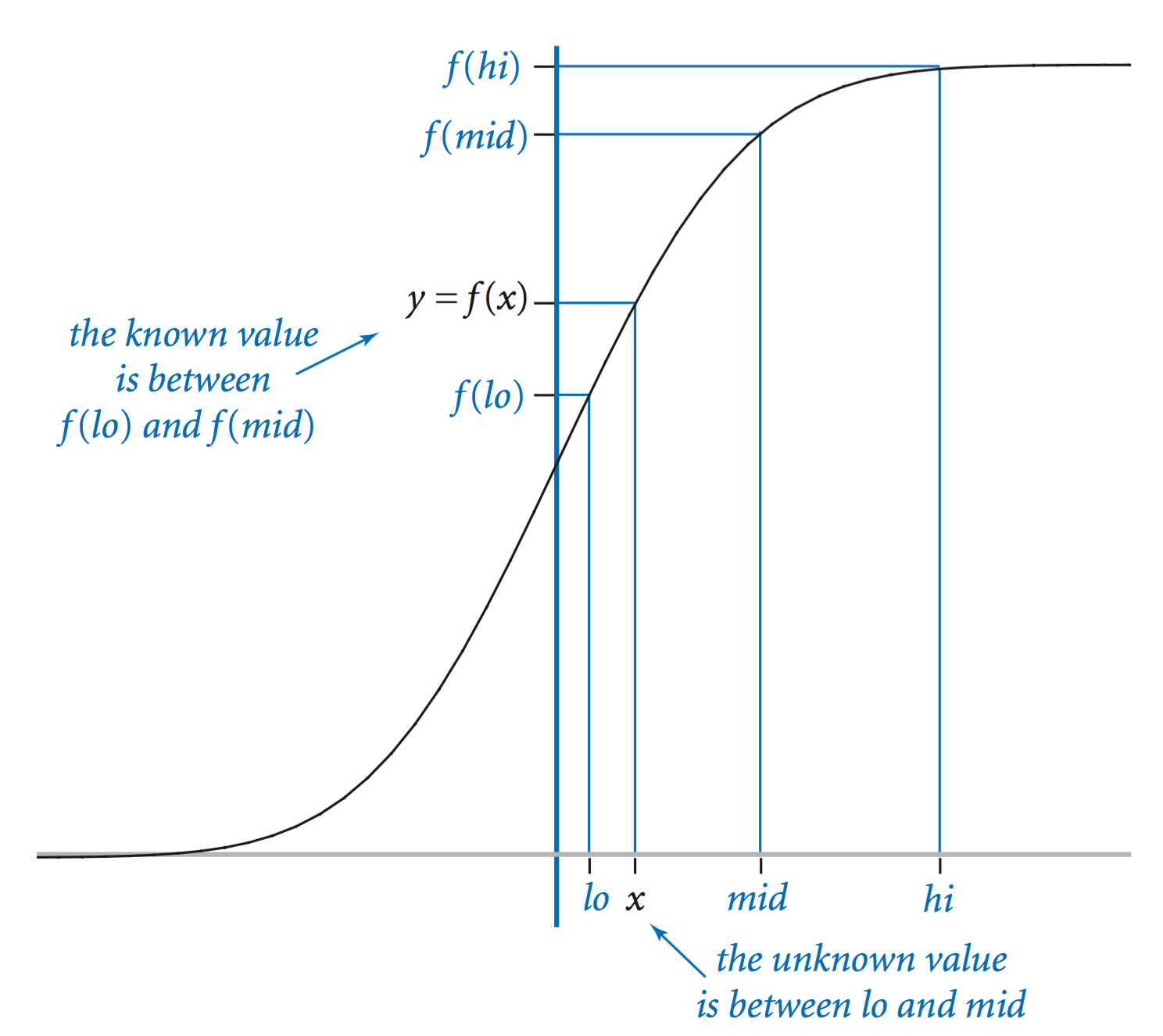• Inverting an increasing function f(x). Given a value y, our task is to find a value x such that f(x) = y. We start with an interval (lo, hi) known to contain x and use the following recursive strategy:
• Compute mid = lo + (hilo) / 2
• Base case: If (hilo) is less than δ, then return mid as an estimate of x
• Recursive step: otherwise, test whether f(mid) > y. If so, look for x in (lo, mid); if not look for x in (mid, hi).

The inverseCDF() method in Gaussian.java implements this strategy for the Gaussian cumulative density function Φ. In this context, binary search is often called bisection search.

•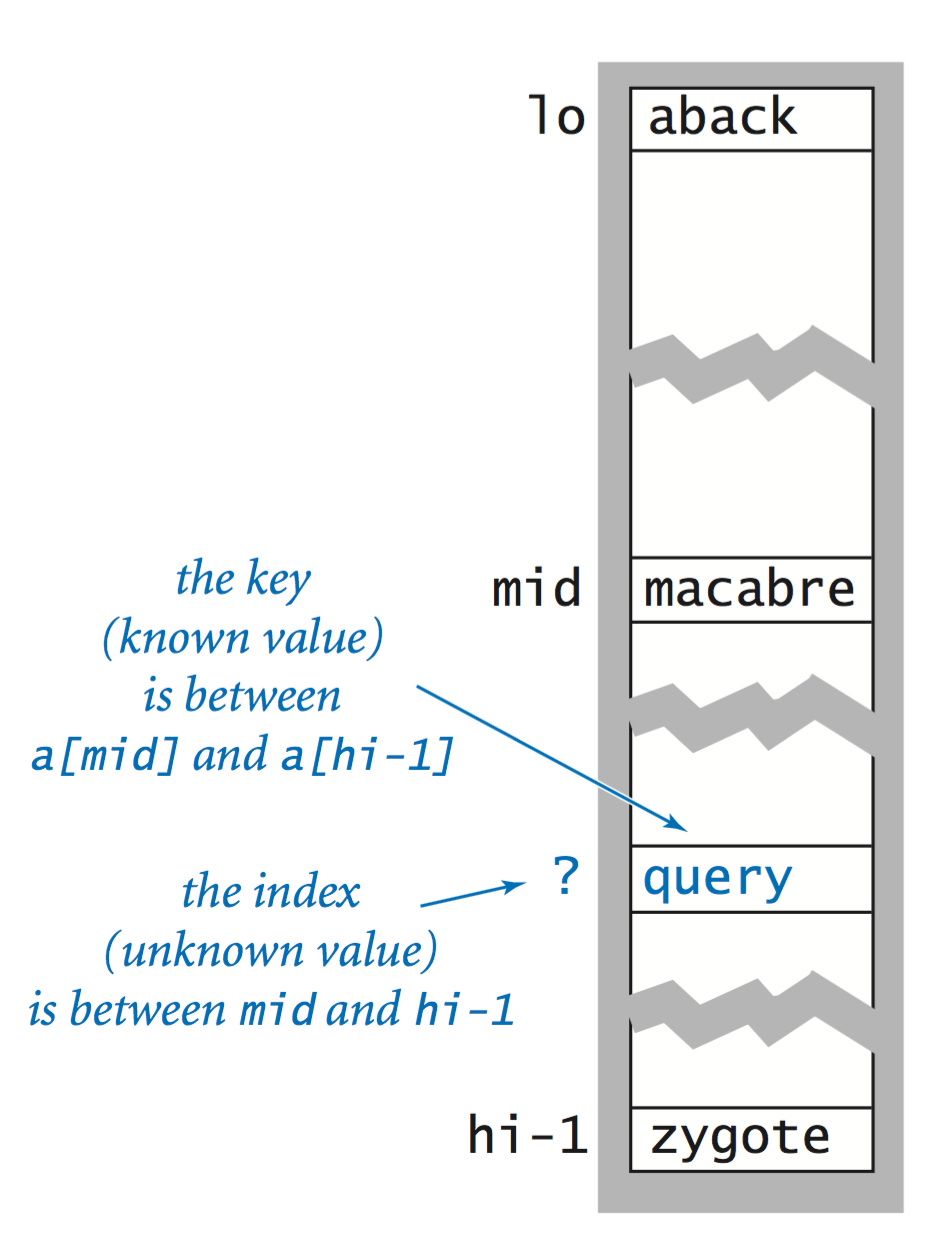• Binary search in a sorted array. One of the most important uses of binary search is to find an item in a sorted array. To do so, look at the array element in the middle. If it contains the item you are seeking, you are done; otherwise, you eliminate either the subarray before or after the middle element from consideration and repeat. BinarySearch.java is an implementation of this algorithm.

## Insertion sort.

Insertion sort is a brute-force sorting algorithm that is based on a simple method that people often use to arrange hands of playing cards: Consider the cards one at a time and insert each into its proper place among those already considered (keeping them sorted). The following code mimics this process in a Java method that sorts strings in an array:
 public static void sort(String[] a) { int n = a.length; for (int i = 1; i < n; i++) { for (int j = i; j > 0; j--) { if (a[j-1].compareTo(a[j]) > 0) exch(a, j, j-1); else break; } } } 
At the beginning of each iteration of the outer for loop, the first i elements in the array are in sorted order; the inner for loop moves a[i] into its proper position in the array by exchanging it with each large value to its left, moving from right to left, until it reaches its proper position. Here is an example when i is 6: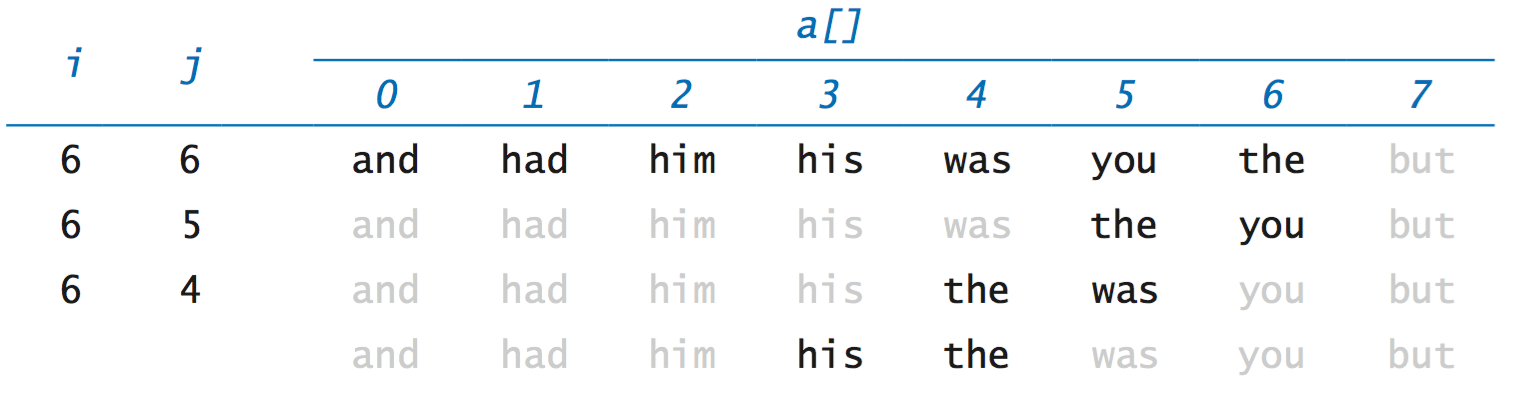This process es executed first with i equal to 1, then 2, then 3, and so forth, as illustrated in the following trace.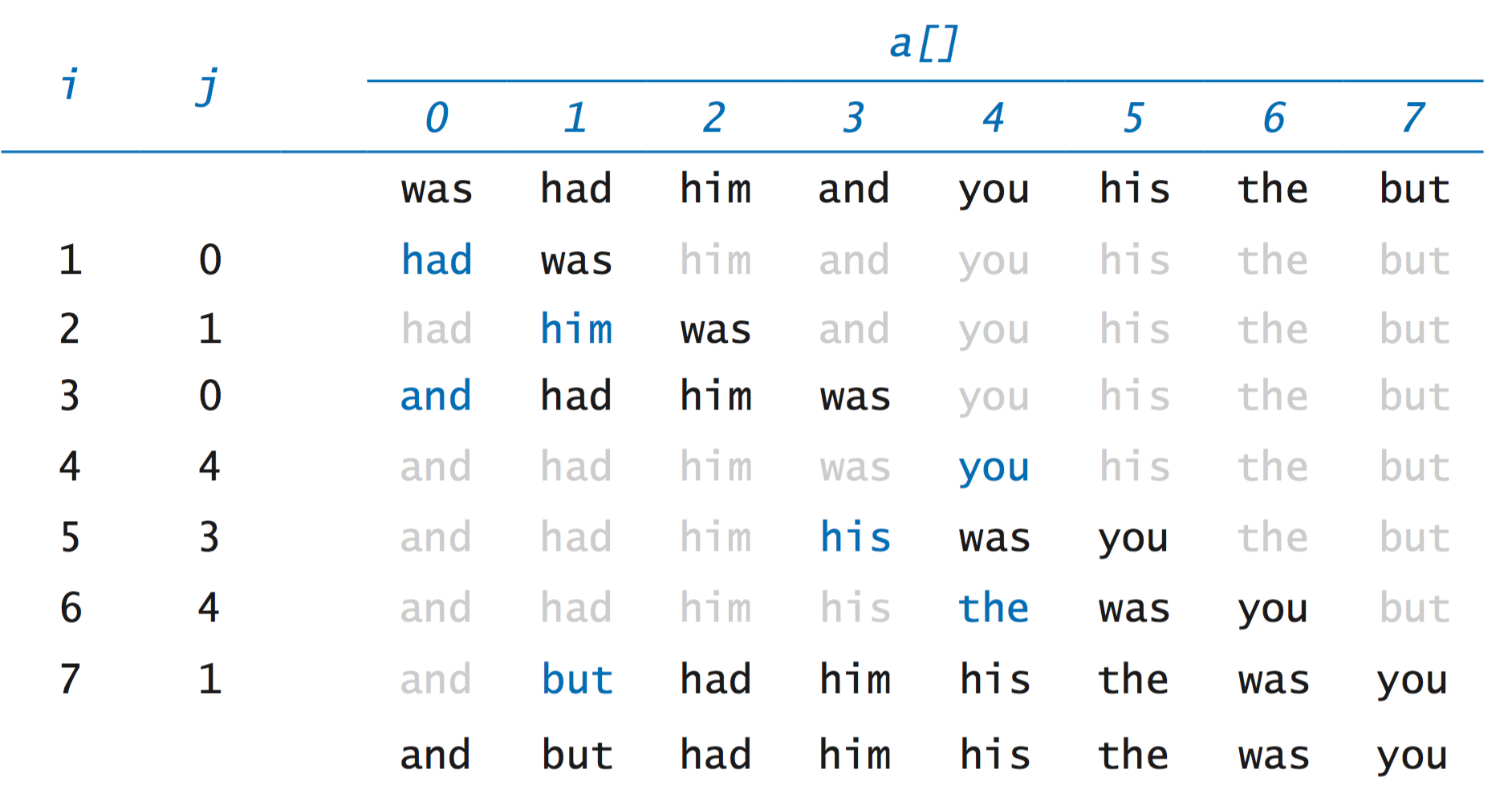• Analysis of running time. The inner loop of the insertion sort code is within a double nested for loop, which suggests that the running time is quadratic, but we cannot immediately draw this conclusion because of the break.
• Best case. When the input array is already in sorted order, the total number of compares in ~ n and the running time is linear.
• Worst case. When the input is reverse sorted, the number of compares is ~ 1/2 n2 and the running time is quadratic.
• Average case. When the input is randomly ordered, the expected number of compares is ~ 1/4 n2 and the running time is quadratic.
• Sorting other types of data. We want to be able to sort all types of data, not just strings. For sorting objects in an array, we need only assume that we can compare two elements to see whether the first is bigger than, smaller than, or equal to the second. Java provides the Comparable interface for this purpose. Insertion.java implements insertion sort so that it sorts arrays of Comparable objects.
• Empirical analysis. InsertionTest.java tests our hypothesis that insertion sort is quadratic for randomly-ordered arrays.

## Mergesort.

To develop a faster sorting method, we use a divide-and-conquer approach to algorithm design that every programmer needs to understand. To mergesort an array, we divide it into two halves, sort the two halves independently, and then merge the results to sort the full array. To sort a[lo, hi), we use the following recursive strategy:
• Base case: If the subarray length is 0 or 1, it is already sorted.
• Reduction step: Otherwise, compute mid = lo + (hi - lo) / 2, recursively sort the two subarrays a[lo, mid) and a[mid, hi), and merge them to produce a sorted result.Merge.java is an implementation of this strategy. Here is a trace of the contents of the array during a merge.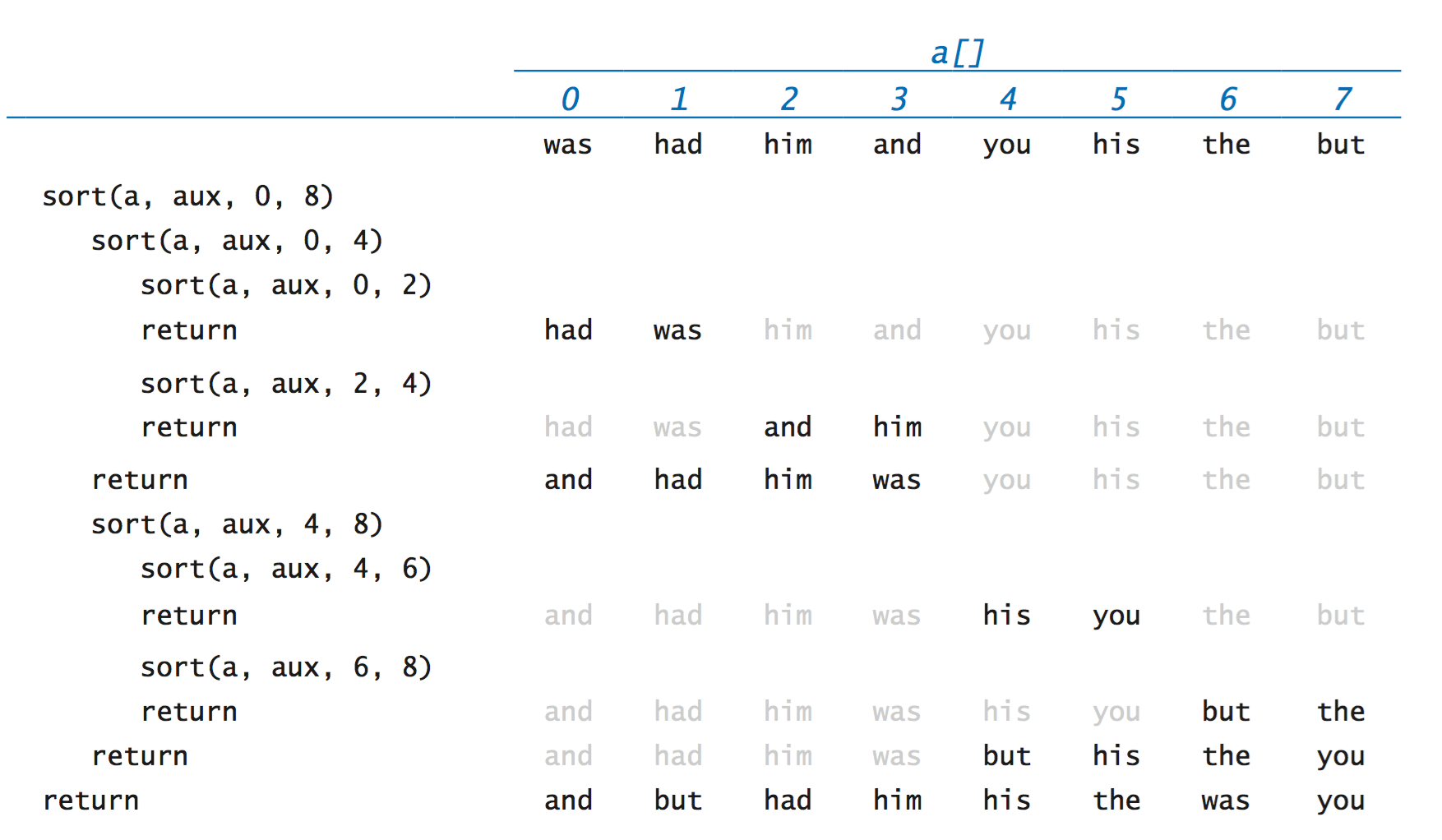• Analysis of running time. In the worst case, mergesort makes between ~ 1/2 n lg n and ~ n lg n compares and the running time is linearithmic. See the book for for details.
• Quadratic–linearithmic chasm. The difference between n2 and n lg n makes a huge difference in practical applications.
• Divide-and-conquer algorithms. The same basic approach is effective for many important problems, as you will learn if you take a course on algorithm design.
• Reduction to sorting. A problem A reduces to a problem B if we can use a solution to B to solve A. For example, consider the problem of determining whether the elements in an array are all different. This problem reduces to sorting because we can sort the array, the make a linear pass through the sorted array to check whether any entry is equal to the next (if not, the elements are all different.)

## Frequency counts.

FrequencyCount.java reads a sequence of strings from standard input and then prints a table of the distinct values found and the number of times each was found, in decreasing order of the frequencies. We accomplish this by two sorts.
• Computing the frequencies. Our first step is to sort the strings on standard input. In this case, we are not so much interested in the fact that the strings are put into sorted order, but in the fact that sorting brings equal strings together. If the input is
 to be or not to be to 
then the result of the sort is
 be be not or to to to 
with equal strings like the three occurrences of to brought together in the array. Now, with equal strings all together in the array, we can make a single pass through the array to compute all the frequencies. The Counter.java data type that we considered in Section 3.3 is the perfect tool for the job.
• Sorting the frequencies. Next, we sort the Counter objects. We can do so in client code without any special arrangements because Counter implements the Comparable interface.
• Zipf's law. The application highlighted in FrequencyCount.java is elementary linguistic analysis: which words appear most frequently in a text? A phenomenon known as Zipf's law says that the frequency of the ith most frequent word in a text of m distinct words is proportional to 1/i.

#### Exercises

1. Write a filter Dedup.java that reads strings from standard input and prints them on standard output with all duplicates removed (in sorted order).

#### Creative Exercises

This list of exercises is intended to give you experience in developing fast solutions to typical problems. Think about using binary search, mergesort, or devising your own divide-and-conquer algorithm. Implement and test your algorithm.

1. Integer sort. Write a linear-time filter IntegerSort.java that reads from standard input a sequence of integers that are between 0 and 99 and prints to standard output the same integers in sorted order. For example, presented with the input sequence
 98 2 3 1 0 0 0 3 98 98 2 2 2 0 0 0 2 
your program should print the output sequence
 0 0 0 0 0 0 1 2 2 2 2 2 3 3 98 98 98 
2. Three sum. Given an array of n integers, design an algorithm to determine whether any three of them sum to 0. The order of growth of the running time of your program should be n2 log n. Extra credit: Develop a program that solves the problem in quadratic time.

Solution: ThreeSumDeluxe.java.

3. Quicksort. Write a recursive program Quick.java that sorts an array of Comparable objects by by using, as a subroutine, the partitioning algorithm described in the previous exercise: First, pick a random element v as the partitioning element. Next, partition the array into a left subarray containing all elements less than v, followed by a middle subarray containing all elements equal to v, followed by a right subarray containing all elements greater than v. Finally, recursively sort the left and right subarrays.
4. Reverse domain. Write a program to read in a list of domain names from standard input, and print the reverse domain names in sorted order. For example, the reverse domain of cs.princeton.edu is edu.princeton.cs. This computation is useful for web log analysis. To do so, create a data type Domain.java that implements the Comparable interface, using reverse domain name order.
5. Local minimum in an array. Given an array a[] of n real numbers, design a logarithmic-time algorithm to find a local minimum (an index i such that both a[i-1] < a[i] and a[i] < a[i+1]).

Solution: Query middle value a[n/2], and two neighbors a[n/2 - 1] and a[n/2 + 1]. If a[n/2] is local minimum, stop; otherwise search in half with smaller neighbor.

#### Web Exercises

1. Union of intervals. Given N intervals on the real line, determine the length of their union in O(N log N) time. For example the union of the four intervals [1, 3], [2, 4.5], [6, 9], and [7, 8] is 6.5.
2. Coffee can problem. (David Gries). Suppose you have a coffee can which contains an unknown number of black beans and an unknown number of white beans. Repeat the following process until exactly one bean remains: Select two beans from the can at random. If they are both the same color, throw them both out, but insert another black bean. If they are different colors, throw the black one away, but return the white one. Prove that this process terminates with exactly one bean left. What can you deduce about the color of the last bean as a function of the initial number of black and white beans? Hint: find a useful invariant maintained by the process.
3. Spam campaign. To initiate an illegal spam campaign, you have a list of email addresses from various domains (the part of the email address that follows the @ symbol). To better forge the return addresses, you want to send the email from another user at the same domain. For example, you might want to forge an email from nobody@princeton.edu to somebody@princeton.edu. How would you process the email list to make this an efficient task?
4. Order statistics. Given an array of N elements, not necessarily in ascending order, devised an algorithm to find the kth largest one. It should run in O(N) time on random inputs.
5. Kendall's tau distance. Given two permutations, Kendall's tau distance is the number of pairs out of position. "Bubblesort metric." Give an O(N log N) algorithm to compute the Kendall tau distance between two permutations of size N. Useful in top-k lists, social choice and voting theory, comparing genes using expression profiles, and ranking search engine results.
6. Antipodal points. Given N points on a circle, centered at the origin, design an algorithm that determines whether there are two points that are antipodal, i.e., the line connecting the two points goes through the origin. Your algorithm should run in time proportional to N log N.
7. Antipodal points. Repeat the previous question, but assume the points are given in clockwise order. Your algorithm should run in time proportional to N.
8. Identity. Given an array a[] of N distinct integers (positive or negative) in ascending order. Devise an algorithm to find an index i such that a[i] = i if such an index exists. Hint: binary search.
9. L1 norm. There are N circuit elements in the plane. You need to run a special wire (parallel to the x-axis) across the circuit. Each circuit element must be connected to the special wire. Where should you put the special wire? Hint: median minimizes L1 norm.
10. Finding common elements. Given two arrays of N 64-bit integers, design an algorithm to print out all elements that appear in both lists. The output should be in sorted order. Your algorithm should run in N log N. Hint: mergesort, mergesort, merge. Remark: not possible to do better than N log N in comparison based model.
11. Finding common elements. Repeat the above exercise but assume the first array has M integers and the second has N integers where M is much less than N. Give an algorithm that runs in N log M time. Hint: sort and binary search.
12. Anagrams. Design a O(N log N) algorithm to read in a list of words and print out all anagrams. For example, the strings "comedian" and "demoniac" are anagrams of each other. Assume there are N words and each word contains at most 20 letters. Designing a O(N^2) algorithms should not be too difficult, but getting it down to O(N log N) requires some cleverness.
13. Pattern recognition. Given a list of N points in the plane, find all subset of 3 or more points that are collinear.
14. Pattern recognition. Given a list of N points in the plane in general position (no three are collinear), find a new point p that is not collinear with any pair of the N original points.
15. Search in a sorted, rotated list. Given a sorted list of N integers that has been rotated an unknown number of positions, e.g., 15 36 1 7 12 13 14, design an O(log N) algorithm to determine if a given integer is in the list.
16. Counting inversions. Each user ranks N songs in order of preference. Given a preference list, find the user with the closest preferences. Measure "closest" according to the number of inversions. Devise an N log N algorithm for the problem.
17. Throwing cats from an N-story building. Suppose that you have an N story building and a bunch of cats. Suppose also that a cat dies if it is thrown off floor F or higher, and lives otherwise. Devise a strategy to determine the floor F, while killing O(log N) cats.
18. Throwing cats from a building. Repeat the previous exercise, but devise a strategy that kills O(log F) cats. Hint: repeated doubling and binary search.
19. Throwing two cats from an N-story building. Repeat the previous question, but now assume you only have two cats. Now your goal is to minimize the number of throws. Devise a strategy to determine F that involves throwing cats O(√N) times (before killing them both). This application might occur in practice if search hits (cat surviving fall) are much cheaper than misses (cat dying).
20. Throwing two cats from a building. Repeat the previous question, but only throw O(√F) cats. Reference: ???.
21. Nearly sorted. Given an array of N elements, each which is at most k positions from its target position, devise an algorithm that sorts in O(N log k) time.

Solution 1: divide the file into N/k pieces of size k, and sort each piece in O(k log k) time, say using mergesort. Note that this preserves the property that no element is more than k elements out of position. Now, merge each blocks of k elements with the block to its left.

Solution 2: insert the first k elements into a binary heap. Insert the next element from the array into the heap, and delete the minimum element from the heap. Repeat.

22. Merging k sorted lists. Suppose you have k sorted lists with a total of N elements. Give an O(N log k) algorithm to produce a sorted list of all N elements.
23. Longest common reverse complemented substring. Given two DNA strings, find the longest substring that appears in one, and whose reverse Watson-Crick complement appears in the other. Two strings s and t are reverse complements if t is the reverse of s except with the following substitutions A<->T, C<->G. For example ATTTCGG and CCGAAAT are reverse complements of each other. Hint: suffix sort.
24. Circular string linearization. Plasmids contain DNA in a circular molecule instead of a linear one. To facilitate search in a database of DNA strings, we need a place to break it up to form a linear string. A natural choice is the place that leaves the lexicographically smallest string. Devise an algorithm to compute this canonical representation of the circular string Hint: suffix sort.
25. Find all matches. Given a text string, find all matches of the query string. Hint: combine suffix sorting and binary search.
26. Longest repeated substring with less memory. Instead of using an array of substrings where suffixes[i] refers to the ith sorted suffix, maintain an array of integers so that index[i] refers to the offset of the ith sorted suffix. To compare the substrings represented by a = index[i] and b = index[j], compare the character s.charAt(a) against s.charAt(b), s.charAt(a+1) against s.charAt(b+1), and so forth. How much memory do you save? Is your program faster?
27. Idle time. Suppose that a parallel machine processes n jobs. Job j is processed from sj to tj. Given the list of start and finish times, find the largest interval where the machine is idle. Find the largest interval where the machine is non-idle.
28. Local minimum of a matrix. Given an N-by-N array a of N2 distinct integers, design an O(N) algorithm to find a local minimum: an pair of indices i and j such that a[i][j] < a[i+1][j], a[i][j] < a[i][j+1], a[i][j] < a[i-1][j], and a[i][j] < a[i][j-1].
29. Monotone 2d array. Give an n-by-n array of elements such that each row is in ascending order and each column is in ascending order, devise an O(n) algorithm to determine if a given element x in the array. You may assume all elements in the n-by-n array are distinct.
30. 2D maxima. Given a set of n points in the plane, point (xi, yi) dominates (xj, yj) if xi > xj and yi > yj. A maxima is a point that is not dominated by any other point in the set. Devise an O(n log n) algorithm to find all maxima. Application: on x-axis is space efficiency, on y-axis is time efficiency. Maxima are useful algorithms. Hint: sort in ascending order according to x-coordinate; scan from right to left, recording the highest y-value seen so far, and mark these as maxima.
31. Compound words. Read in a list of words from standard input, and print out all two-word compound words. If after, thought, and afterthought are in the list, then afterthought is a compound word. Note: the components in the compound word need not have the same length.
32. Smith's rule. The following problem arises in supply chain management. You have a bunch of jobs to schedule on a single machine. (Give example.) Job j requires p[j] units of processing time. Job j has a positive weight w[j] which represents its relative importance - think of it as the inventory cost of storing the raw materials for job j for 1 unit of time. If job j finishes being processed at time t, then it costs t * w[j] dollars. The goal is to sequence the jobs so as to minimize the sum of the weighted completion times of each job. Write a program SmithsRule.java that reads in a command line parameter N and a list of N jobs specified by their processing time p[j] and their weight w[j], and output an optimal sequence in which to process their jobs. Hint: Use Smith's rule: schedule the jobs in order of their ratio of processing time to weight. This greedy rule turns out to be optimal.
33. Sum of four primes. The Goldbach conjecture says that all positive even integers greater than 2 can be expressed as the sum of two primes. Given an input parameter N (odd or even), express N as the sum of four primes (not necessarily distinct) or report that it is impossible to do so. To make your algorithm fast for large N, do the following steps:
1. Compute all primes less than N using the Sieve of Eratosthenes.
2. Tabulate a list of sums of two primes.
3. Sort the list.
4. Check if there are two numbers in the list that sum to N. If so, print out the corresponding four primes.
34. Typing monkeys and power laws. (Michael Mitzenmacher) Suppose that a typing monkey creates random words by appending each of 26 possible letter with probability p to the current word, and finishes the word with probability 1 - 26p. Write a program to estimate the frequency spectrum of the words produced.
35. Typing monkeys and power laws. Repeat the previous exercise, but assume that the letters a-z occur proportional to the following probabilities, which are typical of English text.

CHAR FREQ   CHAR FREQ   CHAR FREQ   CHAR FREQ   CHAR FREQ
A 8.04 G 1.96 L 4.14 Q 0.11 V 0.99
B 1.54 H 5.49 M 2.53 R 6.12 W 1.92
C 3.06 I 7.26 N 7.09 S 6.54 X 0.19
D 3.99 J 0.16 O 7.60 T 9.25 Y 1.73
E 12.51 K 0.67 P 2.00 U 2.71 Z 0.09
F 2.30

36. Binary search. Justify why the following modified version of binarySearch() works. Prove that if the key is in the array, it correctly returns the smallest index i such that a[i] = key; if the key is not in the array, it returns -i where i is the smallest index such that a[i] > key.
 // precondition array a in ascending order public static int binarySearch(long[] a, long key) { int bot = -1; int top = a.length; while (top - bot > 1) { int mid = bot + (top - bot) / 2; if (key > a[mid]) bot = mid; else top = mid; } if (a[top] == key) return top; else return -top - 1; } 
Answer. The while loop invariant says top >= bot + 2. This implies bot < mid < top. Hence length of interval strictly decreases in each iteration. While loop also maintains the invariant: a[bot] < key <= a[top], with the contention that a[-1] is -infinity and a[N] is +infinity.
37. Range search. Given a database of all tolls collected in NJ road system in 2006, devise a scheme to answer queries of the form: extract sum of all tolls collected in a given time interval. Use a Toll data type that implements the Comparable interface, where the key is the time that the toll was collected.

Hint: sort by time, compute a cumulative sum of the first i tolls, then use binary search to find the desired interval.

38. Longest repeated substrings. Modify LRS.java to find all longest repeated substrings.
39. Non-recursive binary search. Write a non-recursive version of binary search.
 public static int binarySearch(long[] a, long key) { int bot = 0; int top = a.length - 1; while (bot <= top) { int mid = bot + (top - bot) / 2; if (key < a[mid]) top = mid - 1; else if (key > a[mid]) bot = mid + 1; else return mid; } return -1; } 
40. Two sum to x. Given a sorted list of N integers and a target integer x, determine in O(N) time whether there are any two that sum to exactly x.

Hint: maintain an index lo = 0 and hi = N-1 and compute a[lo] + a[hi]. If the sum equals x, you are done; if the sum is less than x, decrement hi; if the sum is greater than x, increment lo. Be careful if one (or more) of the integers are 0.

41. Zero of a monotonic function. Let f be a monotonically increasing function with f(0) < 0 and f(N) > 0. Find the smallest integer i such that f(i) > 0. Devise an algorithm that makes O(log N) calls to f().

Hint: assuming we know N, marinating an interval [lo, hi] such that f[lo] < 0 and f[hi] > 0 and apply binary search. If we don't know N, repeatedly compute f(1), f(2), f(4), f(8), f(16), and so on until you find a value of N such that f(N) > 0.

42. Bitonic max. Let a[] be an array that starts out increasing, reaches a maximum, and then decreases. Design an O(log N) algorithm to find the index of the maximum value.
43. Bitonic search. An array is bitonic if it is comprised of an increasing sequence of integers followed immediately by a decreasing sequence of integers. Given a bitonic array a of N distinct integers, describe how to determine whether a given integer is in the array in O(log N) steps. Hint: find the maximum, then binary search in each piece.
44. Median in two sorted arrays. Given two sorted arrays of size N1 and N2, find the median of all elements in O(log N) time where N = N1 + N2. Hint: design a more general algorithm that finds the kth largest element for any k. Compute the median element in the large of the two lists and; throw away at least 1/4 of the elements and recur.
45. Element distinctness. Give an array of N long integers, devise an O(N log N) algorithm to determine if any two are equal. Hint: sorting brings equal values together.
46. Duplicate count. Give a sorted array of N elements, possibly with duplicates, find the index of the first and last occurrence of k in O(log N) time. Give a sorted array of N elements, possibly with duplicates, find the number of occurrences of element k in O(log N) time. Hint: modify binary search.
47. Common element. Write a static method that takes as argument three arrays of strings, determines whether there is any string common to all three arrays, and if so, returns one such string. The running time of your method should be linearithmic in the total number of strings.

Hint: sort each of the three lists, then describe how to do a "3-way" merge.

48. Longest repeated substring. Write a program LRS.java to to find the longest repeated substring in a string. Find the longest repeated substring in your favorite book.
49. Add code to LRS.java to make it print indices in the original string where the longest repeated substring occurs.
50. Longest common substring. Write a static method that finds the longest common substring of two given strings s and t.

Hint: Suffix sort each string. Then merge the two sorted suffixes together.

51. Longest repeated, non-overlapping string. Modify LRS.java to find the longest repeated substring that does not overlap.
52. Rhyming words. Write a program Rhymer.java that tabulates a list that you can use to find words that rhyme. Use the following approach:
• Read in a dictionary of words into an array of strings.
• Reverse the letters in each word (confound becomes dnuofnoc, for example).
• Sort the resulting array.
• Reverse the letters in each word back to their original order.

For example, confound is adjacent to words such as astound and surround in the resulting list.

Scientific example of sorting. Google display search results in descending order of "importance", a spreadsheet displays columns sorted by a particular field, Matlab sorts the real eigenvalues of a symmetric matrix in descending order. Sorting also arises as a critical subroutine in many applications that appear to have nothing to do with sorting at all including: data compression (see the Burrows-Wheeler programming assignment), computer graphics (convex hull, closest pair), computational biology (longest common substring discussed below), supply chain management (schedule jobs to minimize weighted sum of completion times), combinatorial optimization (Kruskal's algorithm), social choice and voting (Kendall's tau distance), Historically, sorting was most important for commercial applications, but sorting also plays a major role in the scientific computing infrastructure. NASA and the fluids mechanics community use sorting to study problems in rarefied flow; these collision detection problems are especially challenging since they involve ten of billions of particles and can only be solved on supercomputers in parallel. Similar sorting techniques are used in some fast N-body simulation codes. Another important scientific application of sorting is for load balancing the processors of a parallel supercomputers. Scientists rely on clever sorting algorithm to perform load-balancing on such systems.